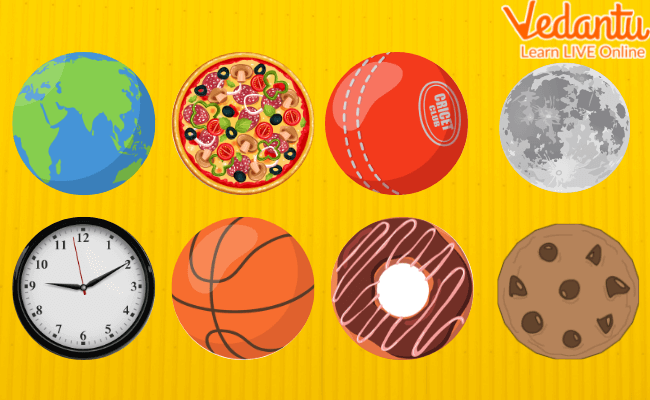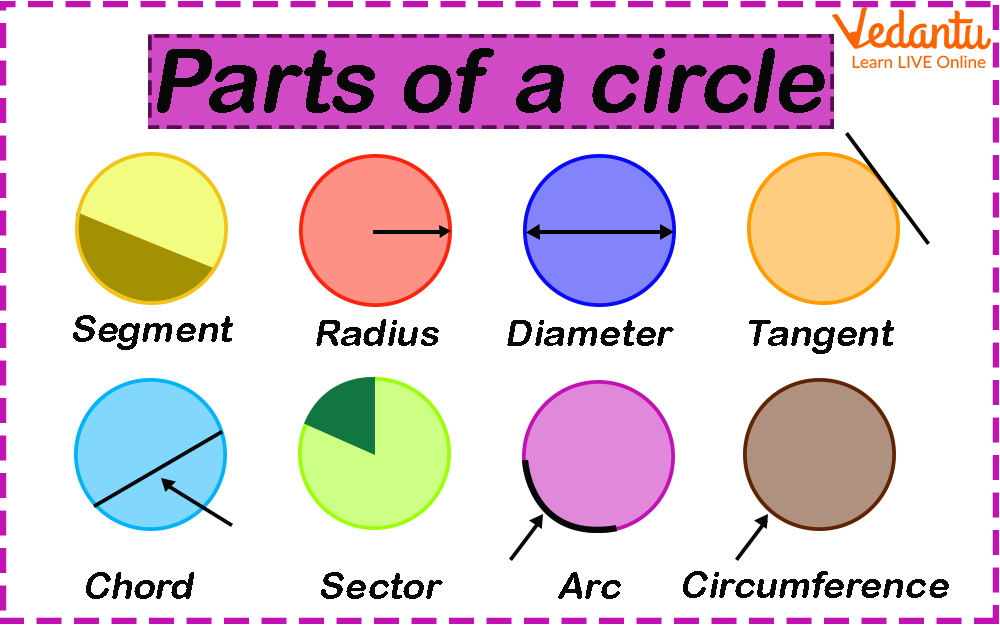Courses
Courses for Kids
Free study material
Free LIVE classes
More

# Circles for Kids - Definitions and PropertiesLIVE
Join Vedantu’s FREE Mastercalss

## What is a Circle?

You see different shapes in your daily life. Do you know what is the shape of a ring, or a coin, or that of the sun and the moon? Yes! It is a circle. A circle is a round shape. All points present on its boundary, a curved line, are placed at an equal distance from its centre. It is a two-dimensional shape. In your day-to-day life, you must have come across several objects that are in the shape of a circle.

For example, a ball, a dish, a clock, a doughnut, etc. Check the image below to know some objects that are in the shape of a circle.Shape of a Circle

## Parts of Circle

The parts of a circle are shown in the below picture.Parts of a Circle

1. Radius: A radius of a circle is a line drawn from the centre of the circle to any point on the circle edge. Letter ‘R’ is generally used to show the radius of the circle.

2. Circumference: Circumference of the circle is defined as the boundary of the circle. We can say that the measure of the boundary of the circle is called the circumference.

3. Chord: In a circle, a chord is a line segment that joins any two points on the circumference of the circle. It divides the circle into two parts. These parts are known as the segments of the circle.

4. Diameter: It is a line segment that passes through the centre of the circle. The endpoints of the diameter lie on the circumference of a circle. The diameter of a circle is double the length of the radius. It is generally represented by the letter ‘D’. Hence 2R=D.

5. Sector: A sector of a circle is the region formed by two of its radii and the arc in between. An angle is subtended at the centre of the circle by a sector.

6. Segment: A segment of a circle is the area that is bounded by an arc and a chord of the circle. There are two types of segments- minor and major. A segment that is made by a minor arc is called a minor segment and a segment that is made by a major arc is called a major segment.

7. Arc: The arc of a circle is a part of its circumference.

8. Tangent: In a circle, a tangent is a straight line that passes by touching the circumference of the circle only at one point. It is important to remember that it does not pass through the circle. The tangent meets the radius of the circle at an angle of 90o.

## Conclusion

In the initial phase of introducing the concept of a circle to students, it is required to teach them the definitions of the parts of a circle. The definitions and concepts discussed above will be of great help for students learning about circles for the first time.

Last updated date: 20th Sep 2023
Total views: 130.2k
Views today: 1.29k

## FAQs on Circles for Kids - Definitions and Properties

1. How do you introduce the shape of a circle to a kid?

Kids come across various objects in their day-to-day lives that make them familiar with different shapes. They may not know the exact name of the particular shapes but they know the shapes of their known objects. For example, if you are teaching circles to a kid, you can give some examples of objects that are in the shape of a circle. This is the easiest way to introduce shapes to your kids.

2. Do circles have an edge?

No, circles do not have edges like squares, rectangles, and other polygons. The boundary of a circle is called circumference which is a curved line. So the circle edge does not exist.